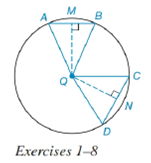Chapter 6.4, Problem 3E### Elementary Geometry for College St...

6th Edition
Daniel C. Alexander + 1 other
ISBN: 9781285195698

#### Solutions

Chapter
Section### Elementary Geometry for College St...

6th Edition
Daniel C. Alexander + 1 other
ISBN: 9781285195698
Textbook Problem
1 views

# In Exercises 1 to 8, use the figure provided.If m C D ⌢ < m A B ⌢ , write an inequality that compares Q M and Q N .To determine

To write:

An inequality that compares QM and QN.

Explanation

Given:

mCD<mAB and the figure given below

Theorem:

In a circle (or in congruent circles) containing two unequal minor arcs, the greater minor arc corresponds to the longer of the chords related to these arcs.

In a circle (or in congruent circles) containing two unequal chords, the shorter chord is at the greater distance from the center of the circle.

Calculation:

Using the theorem.

And since the arcs are unequal i.e. mCD<mAB and from the given figure,

The chord corresponding to arc mCD is CD and the chord corresponding to mAB is AB, we conclude that CD¯<AB¯

### Still sussing out bartleby?

Check out a sample textbook solution.

See a sample solution

#### The Solution to Your Study Problems

Bartleby provides explanations to thousands of textbook problems written by our experts, many with advanced degrees!

Get Started

#### In problems 37-48, compute and simplify so that only positive exponents remain. 42.

Mathematical Applications for the Management, Life, and Social Sciences

#### In Exercises 2340, find the indicated limit. 32. limx2(x2+1)(x24)

Applied Calculus for the Managerial, Life, and Social Sciences: A Brief Approach

#### Evaluate the integral. 042sds

Single Variable Calculus: Early Transcendentals

#### Find if , y = sin 2t.

Study Guide for Stewart's Single Variable Calculus: Early Transcendentals, 8th